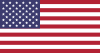### Our website does not fully support your browser.

We've detected that you are using an older version of Internet Explorer. Your commerce experience may be limited. Please update your browser to Internet Explorer 11 or above.

#### We believe this site might serve you best:United States

Language: English

Our website uses functional cookies that do not collect any personal information or track your browsing activity. When you select your country, you agree that we can place these functional cookies on your device.

# Tm for Oligos Calculator

Note: When entering decimal values in concentration fields, please use a decimal point “.” rather than “,” as these calculators use decimal points for input/output of calculations.

OR

### Formula

The most sophisticated Tm calculations take into account the exact sequence and base stacking parameters, not just the base composition(1,2,3).

The equation used is:

${\text{T}}_{\text{m}}=\frac{\mathrm{\Delta }\text{H}\frac{\text{kcal}}{{}^{\circ }\text{C}\phantom{\rule{mediummathspace}{0ex}}×\phantom{\rule{mediummathspace}{0ex}}\text{Mol}}}{\mathrm{\Delta }\text{S + R In ([primer] / 2)}}-273.15{\phantom{\rule{mediummathspace}{0ex}}}^{\circ }\text{C}$$\text{T}_\text{m} = {\Delta\text{H} {\text{kcal}\over ^\circ\text{C}\: \times\: \text{Mol}} \over \Delta\text{S + R In ([primer] / 2)} } - 273.15\:^\circ\text{C}$

$\mathrm{\Delta }$$\Delta$H is the enthalpy of base stacking interactions adjusted for helix initiation factors (3,4).

$\mathrm{\Delta }$$\Delta$S is the entropy of base stacking adjusted for helix initiation factors (3,4) and for the contributions of salts to the entropy of the system (3).

R is the universal gas constant: $1.987\:\text{Cal / }^\circ\text{C} \times \text{Mol}$

Most melting temperature calculations do not take into account the effects of magnesium on helix stability. Therefore, most empirical guidelines used to design experiments will not apply when the magnesium effects are included. We have included the option to consider magnesium in the equation if it is desirable but have not included it in the default setting. Including magnesium will generally raise the theoretical melting temperature by about 5-8°C for oligonucleotides in a 1.5mM Mg2+ solution (5,6).

1. Rychlik, W. and Rhoads, R.E. (1989) Nucl. Acids Res. 17, 8543.
2. Borer P.N. et al. (1974) J. Mol. Biol. 86, 843.
3. SantaLucia, J. (1998) Proc. Nat. Acad. Sci. USA 95, 1460.
4. Allawi, H.T. and SantaLucia, J. Jr. (1997) Biochemistry 36, 10581.
5. von Ahsen N. et al. (1999) Clin. Chem. 45, 2094.
6. Nakano S. et al. (1999) Nucl. Acids Res. 27, 2957.